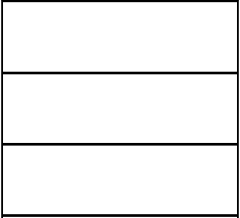# Circumference 46171

Calculate the circumference and area of a rectangle with one side 6 cm long and a diagonal 10 cm long.

o =  28 cm
S =  48 cm2

### Step-by-step explanation:Did you find an error or inaccuracy? Feel free to write us. Thank you!

Tips for related online calculators
The Pythagorean theorem is the base for the right triangle calculator.

#### You need to know the following knowledge to solve this word math problem:

We encourage you to watch this tutorial video on this math problem: